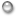# The application of the projection operator method to the derivation of large deviation statistical functions

Nearly half a century has passed since Hazime Mori applied the projection operator method to the analysis of Brownian motion. Later, it was shown that applying this technique to chaotic dynamical systems, we can obtain a closed equation for the two-time correlation functions of dynamical variables exhibiting chaotic dynamics. Such equations contain a memory term, and for this reason, they are difficult to analyze.

However, Hirokazu Fujisaka and collaborators constructed a method for obtaining an approximate form of the two-time correlation function by extending the state space in such a manner that allows for the memory term to be ignored. Further, they developed a technique employing this approximation method with which large deviation statistical functions can be computed.

Unfortunately, Professor Fujisaka died suddenly while working on these problems. However, in accordance with the fundamental ideas he formulated, from among the infinitely many possible ways of extending the state space, that employing a delayed time coordinate has been adopted. The usefulness of the resulting extended state space has been demonstrated through the study of many applications. One clear such example involves the computation of quantities relating to large deviation statistics.

If we attempt to compute such quantities (for example, the rate function) for a random time series (a “signal”) in strict accordance with their definitions, the meaningfulness of the results will be limited by the size of the sample, owing to the fluctuations implied by its finite size — i.e., the finite-size effect. However, if we employ the method introduced by Professor Fujisaka, there is no limitation resulting from the finite-size effect, because instead of determining such quantities as the rate function in strict accordance with their definitions, we obtain approximate forms by approximately solving “evolution equations” that they satisfy.Lecturer Syuji Miyazaki The application of the projection operator method to the derivation of large deviation statistical functions Large fluctuations exhibited by graph networks Chaos analysis of natural languages

ページtopへ▲